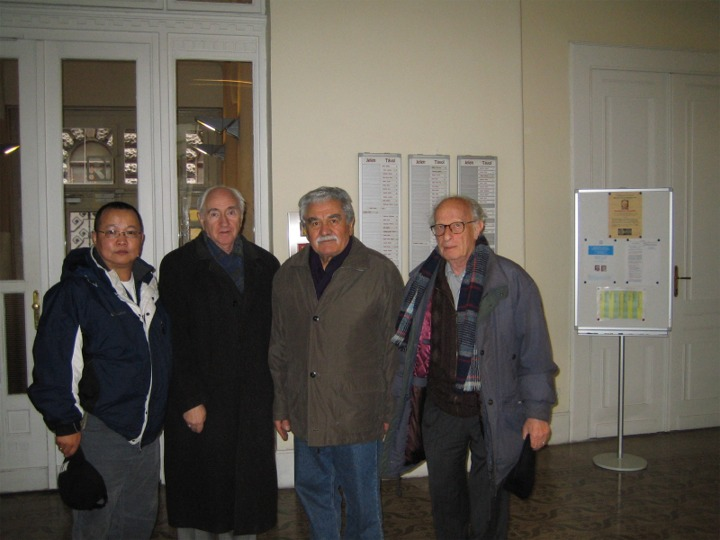# Hurlee Gonchigdanzan

## Professor

Ph.D., Mathematics, University of Cincinnati
M.Sc., Statistics, University of Illinois at Urbana-Champaign
B.Sc., Mathematics, National University of Mongolia

(Hurlee's old webspage)

## Research Interests

Limit Theorems in Probability Theory and Mathematical Statistics, and Stochastic Processes

## Publications

(2019) Almost sure central limit theorems for weighted dependent sequences. Studia Sci. Math. Hung., 56(2), 145-153.

(2011) How much does a Hamiltonian cycle weigh in a complete graph K6? (preprint)

(2010) Almost sure functional limit theorem for the product of partial sums. ESAIM: Probab and Stat., Vol 14, 338 -342.

(2009) How much does a Hamiltonian cycle weigh in complete graphs K4 and K5? ArXiv.org: 0907.5016v2

(2009) with Kosinski, K., On the functional limits for partial sums under stable law. Statist. Probab. Letters, 79(17), 1818 -1822.

(2008) An almost sure limit theorem for the product of partial sums with stable distribution. Statist. Probab. Letters, 78(18), 3170 -3175.

(2006) with Rempala, G., Almost sure limit theorem for the product of partial sums. Applied Math. Letters, 19(2), 191-196.

(2005) On the almost sure limit theorem for the product of U-statistics. Period. Math. Hung., 50(1-2), 149-153.

(2002) with Csáki, E., Almost sure limit theorems for the maximum of stationary Gaussian sequences. Statist. Probab. Letters, 58(2), 195-203

(2002) On the pointwise central limit theorem for strongly mixing and associated random variables. Int. Jour. Math. & Math. Sci., 29(3), 125 -131.

(2001) Almost Sure Limit Theorems. Ph.D. Dissertation, EDT, OhioLINK.

(1997) Almost sure limit theorems for dependent random variables. Studia Sci. Math. Hung., 33, 167-175.

(1996) On the almost sure central limit theorem for φ-mixing random variables. Studia Sci. Math. Hung., 31, 197-202.

(1995) On the almost sure local and global central limit theorem for weakly dependent random variables. Ann. Univ. Sci. Budapest, Sect. Math., 38, 109-126.

(1995) An approximation for logarithmic averages of partial sums of random variables. Period. Math. Hung., 31(3), 189-200

## Presentations

Asymptotic Results in Probability and StatisticsThe conference that honored the 75th birthday of two great Hungarian mathematicians, Csáki Endre and Révész Pál

Rényi Institute of Mathematics, Budapest, Hungary, November 5-7, 2009